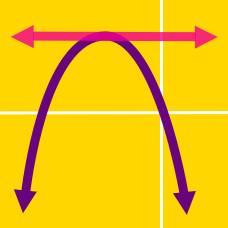Algebra

Completing the Square - Applications

As $x$ ranges over all real values, what is the minimum value of $x^ 2 + 28 x + 313$?

As $x$ ranges over all real values, what is the smallest integer value of

$\frac{ x^2 + 22x + 333} { 11} ?$

Details and assumptions

Note that we are not asking for the value of $x$.

If $\sqrt{ 36 + 16 \sqrt{5} } = a + b \sqrt{c}$, where $a, b$ and $c$ are positive integers and $c$ is not divisible by the square of a prime. Determine $a+b+c$.

The quadratic equation $3x^2+24x-8=0$ can be expressed as $(x-m)^2=n,$ where $m$ and $n$ are constants. What is the value of $m+3n$?

If the solution of the equation $x^2+6x-1=0$ is $x=a \pm \sqrt{b}$, what is $a+b$?

×